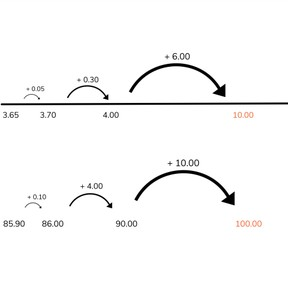Place value- composing decimal numbers to 1, 10, and 100

# Place value- composing decimal numbers to 1, 10, and 100

Place value- composing decimal numbers to 1, 10, and 1008,000 schools use Gynzy92,000 teachers use Gynzy1,600,000 students use Gynzy

## General

Students learn to compose decimals to the whole numbers of 1, 10, and 100. They also practice decomposing the numbers 1, 10, and 100 into decimal numbers.

-5.NBT.B.7

## Relevance

It is important for students to be able to do this to be able to calculate using decimal numbers. This can also be useful for if they are saving money for a particular goal and want to know how much more they need.

## Introduction

Practice composing 100 with whole numbers. Practice with approximate calculations (using one more or less). Next complete the number sequences. The first two number sequences increase by 0.1 and the last two by 0.001. Then you calculate as a class with a number in which you know the ones, tenths, and hundredths: 3.00+0.70 + 0.04.

## Development

When composing to 1, the students should always start by composing to the next tenth (first place after the decimal point) and then to 1. In this case you go from 0.68 to 0.70, an increase of 0.02. Then you compose 1, which means 0.30 more is counted. The total of 0.30 + 0.02 = 0.32. Practice composing to \$1.00. In dragging the coins and bills, you also show the decomposition of the whole 1.
When composing to 10, the students should always compose to the next tenth (first place after the decimal point), then to the next whole number, and then to 10. In this case you go from 3.65 to 3.70, an increase of 0.05. Then you go to 4.00, an increase of 0.30. You then compose 10.00, an increase of 6.00. The total of 0.05 + 0.30 + 6.00= 6.35. You practice this by composing \$10.00. By dragging the coins and bills, you also show the decompositions of the whole 10.00.
To compose to 100 students first compose to the next whole number, then to the next ten and then to 100. In this case you go from 85.90 to 86.00, an increase of 0.10. Then you go to 90.00, an increase of 4.00. You finally compose 100, an increase of 10.00. The total of 0.10 + 4.00 + 10.00 = 14.10. You practice this by composing \$100.00. By dragging coins and bills, you also show the decompositions of the whole 100.00.

To check that students understand composing decimal numbers to 1, 10, and 100, you can ask the following questions:
- Compose 1: 0.2 and...?
- Compose 1: 0.22 and...?
- Compose 10: 5.8 and...?
- Compose 10: 7.99 and...?
- Compose 100: 96.5 and...?
- Compose 100: 92.25 and...?

## Guided practice

Students practice with number bond charts. First with 1, then with 10, and finally with 100.

## Closing

Discuss that it is useful to know how to compose to these numbers if you are saving money, for example to reach \$100. If you already have saved a portion of the money, but want to know how much more you need to save, you are using this skill. When else might you compose decimal numbers? Check that students are able to compose to 1, 10, and 100. The measuring cup has 0.1 liters. How many more must be added to make 1 liter? Ask students to explain their thinking. Then ask how many more pounds the suitcase can weigh. The suitcase weighs 8.3 pounds and the maximum weight is 10 pounds. Finally make a number bond chart in which the students must compose to 1, 10, and 100 with different decimal numbers.

## Teaching tips

Students who have difficulty with this can be supported by the use of manipulatives, like money. Give them an amount and ask them to compose a round number. Have them set the amounts next to each other, so they can see what they had, and what has been added. Ask them to explain the steps they take in their thinking. Good practice with decimals? First compose to a whole number and then go on to a larger number.

## Instruction materials

Play money

### The online teaching platform for interactive whiteboards and displays in schools

• Save time building lessons

• Manage the classroom more efficiently

• Increase student engagement